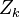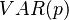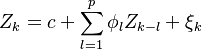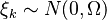# Macroeconomic Scenarios

## Definition

In the context of Stress Testing a Credit Network, Macroeconomic Scenarios are multi-year projections of economic variables.

Macro information comprises of the aggregate, dynamic, economic conditions that can affect the Credit Risk of debtors. The salient feature of macro information that requires to include it as a properly modelled forward-looking element is the empirical fact that

• macro factors do have an influence on credit risk and
• economic states do not evolve as pure random walks but exhibit memory phenomena (auto-regression).

If we ignore current macro conditions in the formation of expectations we may be making a biased estimate. The degree of the bias depends on the degree to which future macro state realizations are uncorrelated with their current value. Hence even if a variable is strongly correlated with the default rate, unless it exhibits autocorrelation, its inclusion to the information set $M_k$ is redundant.

## Usage

Scenarios are typically named to suggest their nature (e.g. Adverse Scenario, Baseline Scenario etc.). Typical macro variables are listed here

## Macroeconomic Factor Processes

A fairly general expression for the process followed by an observable macro factor vector$Z_{k}$ is the standard$VAR(p)$$Z_{k} = c + \sum_{l=1}^{p} \phi_{l} Z_{k-l} + \xi_{k}$

where$\xi_k \sim N(0,\Omega)$.

## Issues and Challenges

The modelling of macroeconomic variables is notoriously difficult